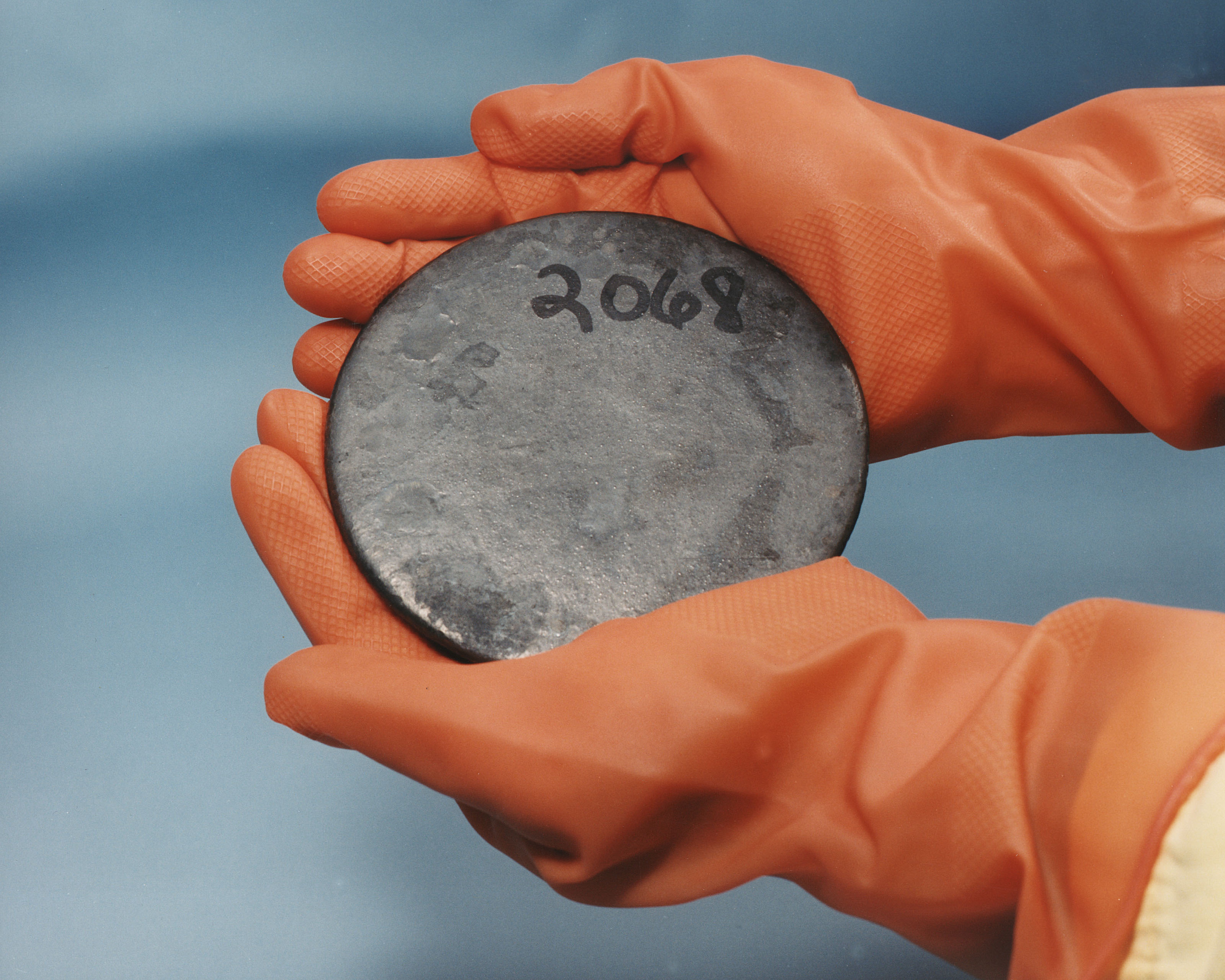# Uranium-239

﻿
Uranium-239

Infobox isotopealternate_names = U-239
symbol =U
mass_number =239
mass =
num_neutrons =147
num_protons =92
abundance = 0 (Artificial)
halflife = 23.45 mins
error_halflife =
background = #fc6
text_color =

decay_product =Neptunium-239
decay_symbol =Np
decay_mass = 239
parent =
parent_symbol =
parent_mass =
parent_decay =
parent2 =
parent2_symbol =
parent2_mass =
parent2_decay =
spin =
excess_energy =
error1 =
binding_energy =
error2 =
decay_mode1 = Beta decay 20%
decay_energy1 = 1.28
decay_mode2 = Beta decay 80%
decay_energy2 = 1.21
decay_mode3 =
decay_energy3 =
decay_mode4 =
decay_energy4 =
Uranium-239 is an isotope of uranium. It is usually produced by exposing uranium-238 to neutron radiation in a nuclear reactor. Uranium-239 has a half-life of about 23.45 minutes and decays into neptunium-239 through beta decay, with a total decay energy of about 1.29 Mev. [CRC Handbook, 57th Ed. p. B-345] . The most common gamma decay at 74.660 kev accounts for the difference in the two major channels of beta emission energy, at 1.28 and 1.21 Mev. [Ibid. p. B-423]

Neptunium-239 further decays to plutonium-239, in a second important step which ultimately produces fissile plutonium-239 (used in weapons and for nuclear power), from uranium-238 in reactors.

Isotope|element=Uranium
lighter=Uranium-238
heavier=Uranium-240
before=Protactinium-239 "'(β-)
after=Neptunium-239 "'(β-)

References

[http://www.euronuclear.org/info/encyclopedia/breeding.htm The European Nuclear Society]

Wikimedia Foundation. 2010.